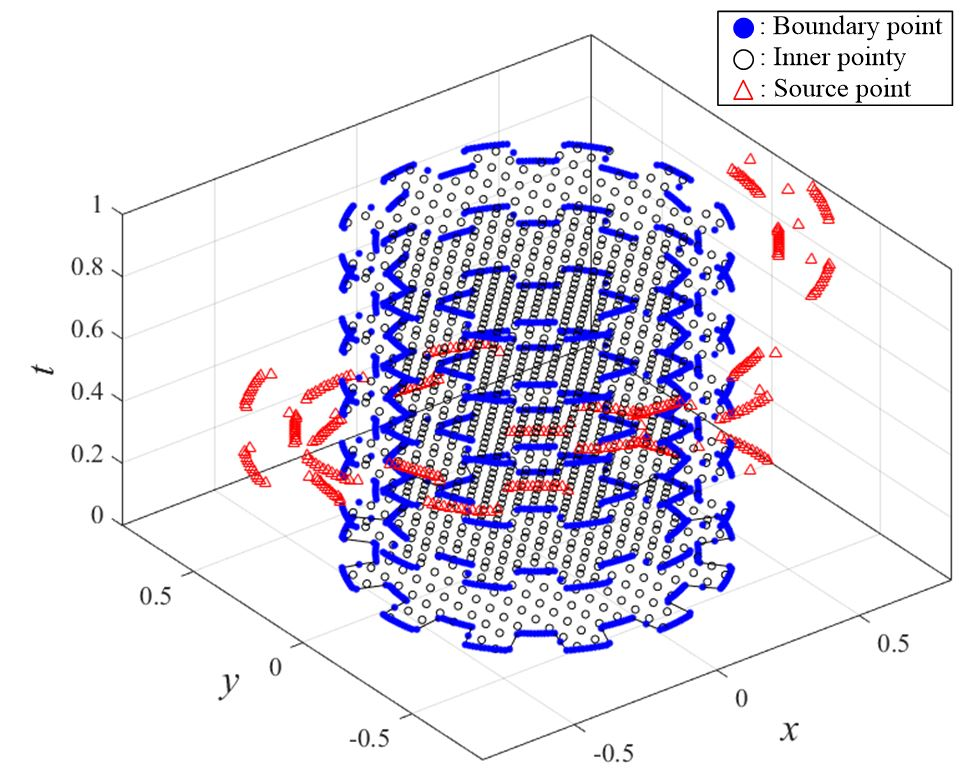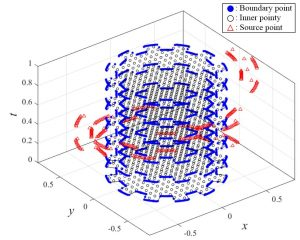# Space–Time Radial Basis Function–Based Meshless Approach for Solving Convection–Diffusion EquationsAbstract:This article proposes a space–time meshless approach based on the transient radial polynomial series function (TRPSF) for solving convection–diffusion equations. We adopted the TRPSF as the basis function for the spatial and temporal discretization of the convection–diffusion equation. The TRPSF is constructed in the space–time domain, which is a combination of n–dimensional Euclidean space and time into an n + 1–dimensional manifold. Because the initial and boundary conditions were applied on the space–time domain boundaries, we converted the transient problem into an inverse boundary value problem. Additionally, all partial derivatives of the proposed TRPSF are a series of continuous functions, which are nonsingular and smooth. Solutions were approximated by solving the system of simultaneous equations formulated from the boundary, source, and internal collocation points. Numerical examples including stationary and nonstationary convection–diffusion problems were employed. The numerical solutions revealed that the proposed space–time meshless approach may achieve more accurate numerical solutions than those obtained by using the conventional radial basis function (RBF) with the time-marching scheme. Furthermore, the numerical examples indicated that the TRPSF is more stable and accurate than other RBFs for solving the convection–diffusion equation.

Keywords: space–time; transient radial basis function; convection–diffusion equation; meshless; radial polynomials```Abstract: https://www.mdpi.com/2227-7390/8/10/1735
PDF Version: https://www.mdpi.com/2227-7390/8/10/1735/pdf```

]]>